# Continuity Chapter Exam

Exam Instructions:

Choose your answers to the questions and click 'Next' to see the next set of questions. You can skip questions if you would like and come back to them later with the yellow "Go To First Skipped Question" button. When you have completed the practice exam, a green submit button will appear. Click it to see your results. Good luck!

### Page 1

#### Question 1 1. What are the regions of continuity in this graph of f(x)?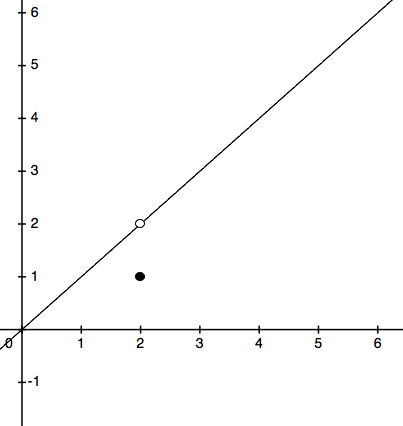#### Question 2 2. For the following function, does the intermediate value theorem apply between x = -2 and x = 2?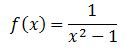#### Question 3 3. What are the regions of continuity given the following?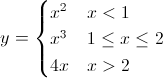### Page 3

#### Question 11 11. Given the values of the continuous function f(x) below, how many solutions will there be to f(x)=4.1?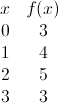#### Question 12 12. Consider the function below. According to the intermediate value theorem, is there a solution to f(x) = 0 for a value of x between -5 and 5?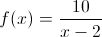#### Question 14 14. What region is the following continuous over?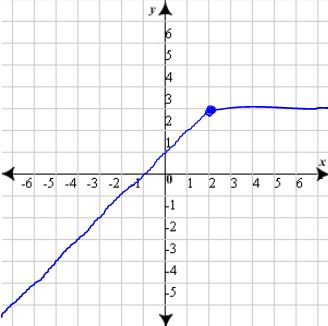### Page 4

#### Question 16 16. Consider the equation below. Calculate f(0), f(1) and f(2). Given that information, how many solutions are there to f(x)=-1?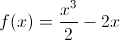#### Question 17 17. What are the regions of continuity for y defined as?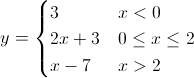#### Question 18 18. What kind of discontinuity does this function have?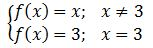#### Question 19 19. Consider the function f(x) below. Considering the intermediate value theorem and the vertical asymptote at x=0, is the following statement true or false? For any number C greater than 0, there is a positive value of x that satisfies the equation f(x)=C.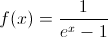### Page 5

#### Question 21 21. Given y below and the intermediate value theorem, how many times will y = 0 between x = 0 and x = π?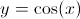#### Question 22 22. In the following graph, is the function continuous over the region between x = -8 and x = -2?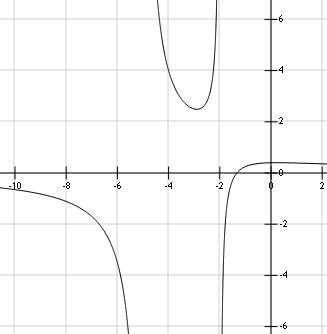#### Question 23 23. Which of the following continuous functions will have at least one solution to the following equation?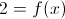#### Question 25 25. Given the function below, in what region will f(x) = 0?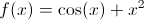### Page 6

#### Question 27 27. What kind of discontinuity is shown in this graph?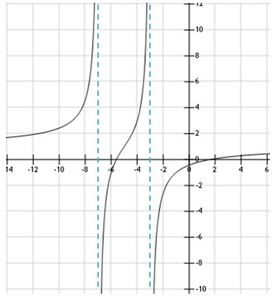#### Question 29 29. What are the regions of continuity in the graph of f(x) below?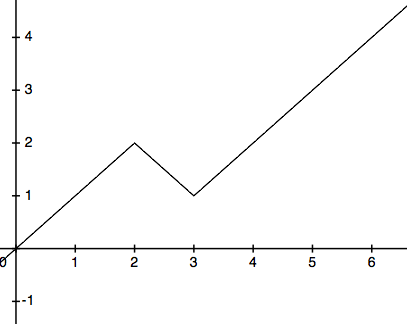#### Question 30 30. How many discontinuities are in this function?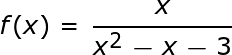#### Continuity Chapter Exam Instructions

Choose your answers to the questions and click 'Next' to see the next set of questions. You can skip questions if you would like and come back to them later with the yellow "Go To First Skipped Question" button. When you have completed the practice exam, a green submit button will appear. Click it to see your results. Good luck!

Support